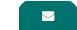## COMC加拿大国手选拔赛真题数据分析报告

COMC通常在每年9月1日开放报名，加拿大和美洲（北/南美洲时区）比赛时间为2018年11月8日，其他国家比赛时间为11月9日。

COMC要求参赛选手年龄在19岁以下，具有加拿大公民或居民身份的全职学生都可以报名参加(即在校高中生)。学生不可以自己报名，必须通过所在学校的数学系或老师报名。海外居住的加拿大人，符合以上要求的也可以报名。其他国家的代表队也可以报名参加比赛，以增加比赛的国际竞争力，但不会作为加拿大奥林匹克代表队的候选人。

COMC满分80分，要求答题者写出步骤，有点类似于滑铁卢的Fryer、Galois、Hypatia和Euclid,不同于AMC和滑铁卢系列的其他多项选择题比赛。

COMC整个试卷分成三个部分：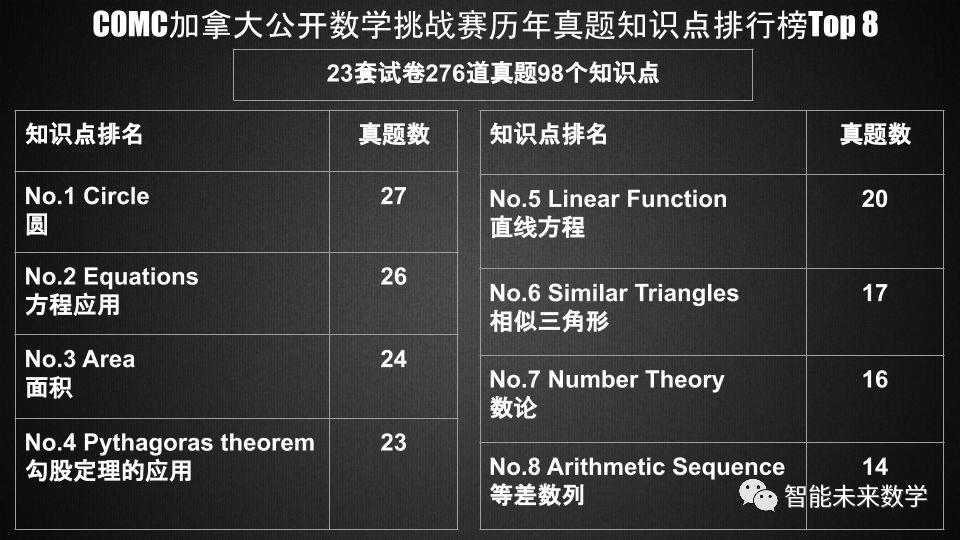2001年的第12题；

2002年的第12题；

2003年的第11题；

2005年的第12题；

2010年的第11题；

2012年的第11题......

COMC几乎把解析几何的直线方程应用锁定在了第9题和第10题，其中有14年的第9题和第10题都是直线方程。

1997年的第9题；

1998年的第9题；

1999年的第9题；

2000年的第9题；

2001年的第9题；

2002年的第9题；

2004年的第9题；

2005年的第9题；

2006年的第10题；

2009年的第10题；

2010年的第10题；

2011年的第9题；

2014年的第10题；

2015年的第10题；

......

2005年的第11题；

2009年的第12题；

2010年的第12题；

2013年的第11题；

2015年的第11题；

2016年的第11题；

2017年的第12题；

2017年的第9题；

2018年的第12题；

......

COMC的数据分析报告，终于让可爱的圆挽回了一些面子。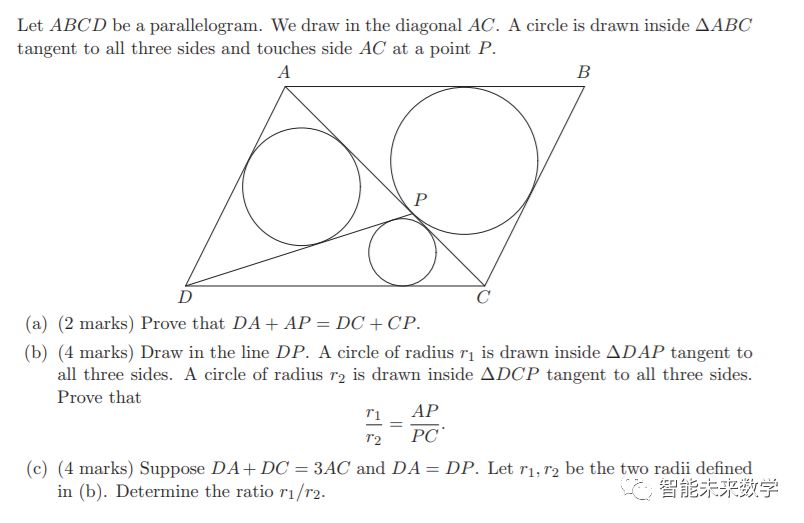(a)求证：DA+AP=DC+CP;

(c)假设DA+DC=3AC并且DA=DP,r1和r2仍然是(b)中的半径，试求：r1/r2;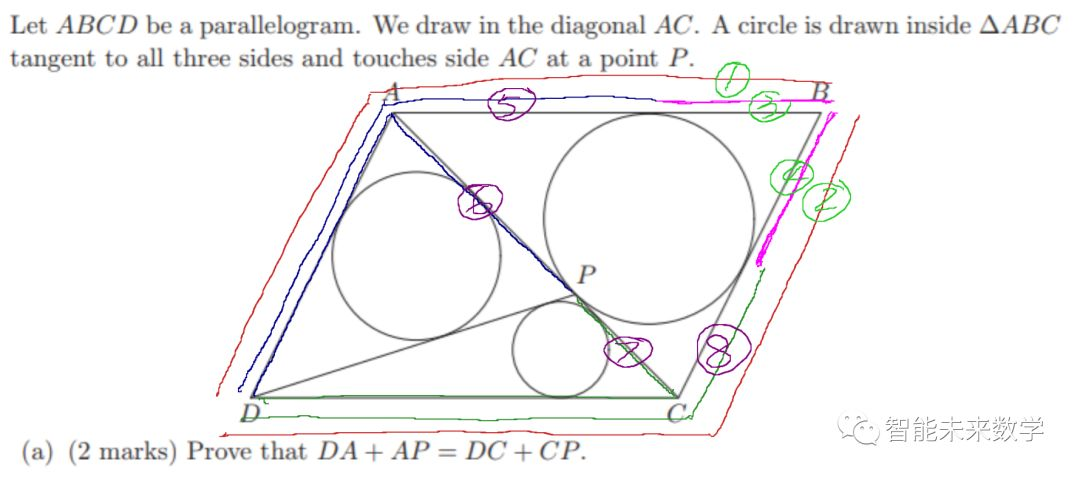(a)证毕。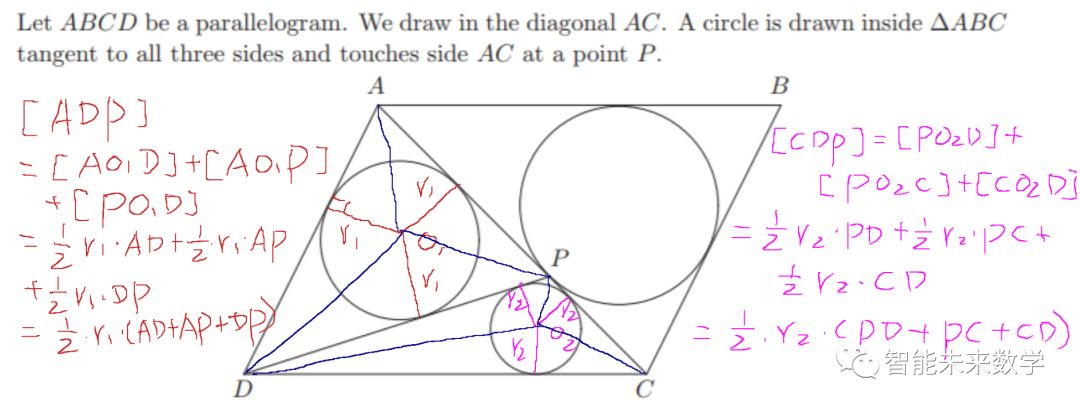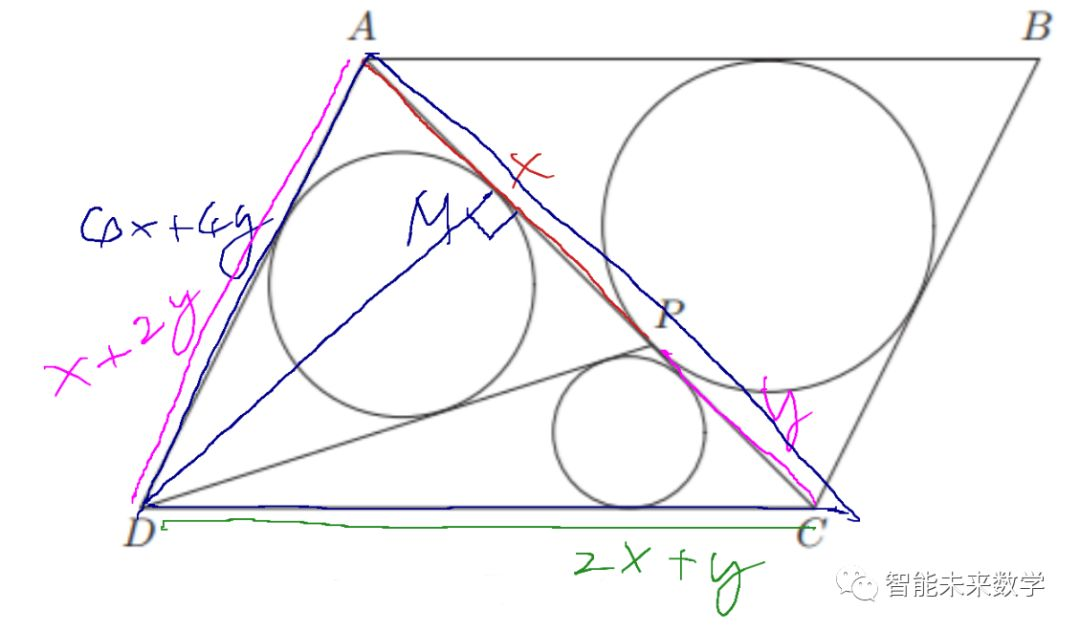(一)根据条件DA=AP,易知DAP是等腰三角形，根据对称性，连接切点M与D得到DM垂直于AC,并且AM=MP;

(二)再利用条件DA+DC=3AC。

DA+AP=DC+CP

=(4x+4y)/2=2x+2y；

(三)通过勾股定理找到x和y的等量关系：

( 2x+y)^2-(x/2+y)^2

=(x+2y)^2-(x/2)^2

x,y都为长度，不能为0，必有3x=4y；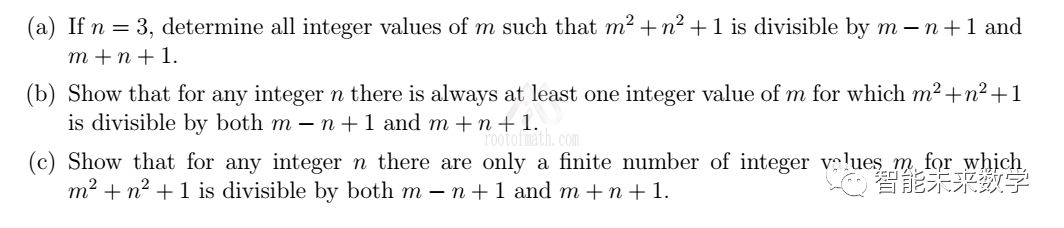(a)如果n=3,找出所有的m使得m^2+n^2+1可以被m-n+1和m+n+1整除；

(b)证明对于任意的整数n，总有至少一个整数m使得m^2+n^2+1可以被m-n+1和m+n+1整除；

(c)证明对于任意的整数n，只存在有限个整数m使得m^2+n^2+1可以被m-n+1和m+n+1整除；

m-2|m^2+10 和 m+4|m^2+10

( 为描述简单，启用符号“|”，是数论符号，表示能够整除，下同)

m^2+10=(m+4)(m-4)+26

m=-30,-17,-6,-5,-3,-2,9,22

(b)此题考察考生因式分解的经验，熟悉平方差公式的同学容易给出：

(n^2+n+1)(n^2-n+1)

=((n^2+1)+n)((n^2+1)-n)

=(n^2+1)^2-n^2

=n^4+n^2+1

=(n^2)^2+n^2+1

m+n-1|m^2+n+1 和 m+n+1|m^2+n+1

(c)仍然是因式分解,不过这里有点技巧，需要把变量m吸入到因式乘积中，让剩下的项与m无关，这样m的范围就被n夹住了：

m^2+n^2+1

=(m+(n-1))(m-(n-1))+(n-1)^2+n^2+1

=(m+(n-1))(m-(n-1))+2(n^2-n+1)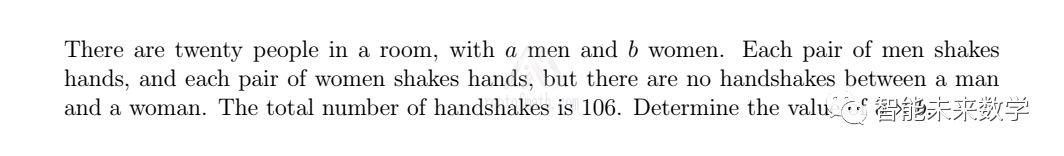a+b=20  (1)

2Ca+2Cb=a(a-1)/2+b(b-1)/2=106 (2)

a^2+b^2=212+(a+b)=232 （3）

( a+b 用(1)中的20代入 )

2(a^2-20a+84)=0

2(a-14)(a-6)=0

AMC美国数学竞赛10年级历年真题数据分析报告

UBC Elmacon数学竞赛历年真题数据分析报告